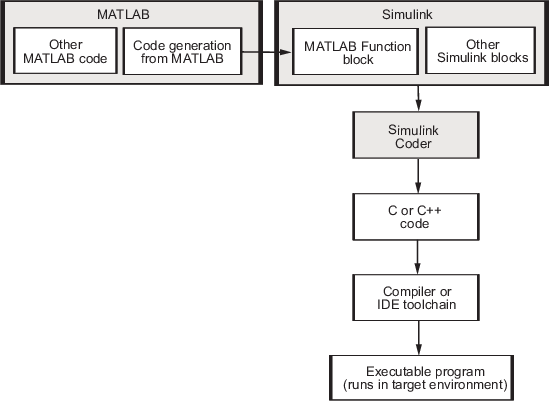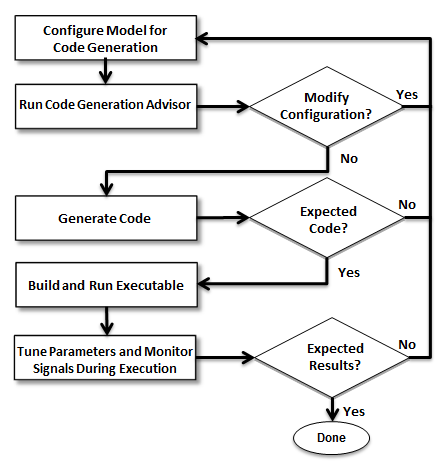Code Generation by Using Simulink Coder

Code Generation Technology

MathWorks® code generation technology produces C or C++ code and executable programs for algorithms. You can write algorithms programmatically by using MATLAB® or graphically in the Simulink® environment. You can generate code for MATLAB functions and Simulink blocks that are useful for real-time and embedded applications. Generated source code and executable programs for floating-point algorithms match the functional behavior of MATLAB code execution and Simulink simulations to a high degree of fidelity. Using the Fixed-Point Designer product, you can generate fixed-point code that provides a bitwise match to model simulation results. Such broad support and high degree of accuracy are possible because code generation is tightly integrated with the MATLAB and Simulink execution and simulation engines. The built-in accelerated simulation modes in Simulink use code generation technology.

Code generation technology and related products provide tooling that you can apply to the V-model for system development. The V-model is a representation of system development that highlights verification and validation steps in the development process. For more information, see Validation and Verification for System Development.

To learn about model design patterns that include Simulink blocks, Stateflow® charts, and MATLAB functions, and map to commonly used C constructs, see Modeling Patterns for C Code (Embedded Coder).

Code Generation Workflow by Using Simulink Coder

Use MathWorks code generation technology to generate standalone C or C++ source code for rapid prototyping, simulation acceleration, and hardware-in-the-loop (HIL) simulation:

• By developing Simulink models and Stateflow charts, and then generating C/C++ code from the models and charts by using the Simulink Coder™ product

• By integrating MATLAB code for code generation in MATLAB Function blocks in a Simulink model, and then generating C/C++ code by using the Simulink Coder product

You can generate code for most Simulink blocks and many MathWorks products. This figure shows the product workflow for code generation by using Simulink Coder. Other products that support code generation, such as Stateflow software, are available.The code generation workflow is a part of the V-model for system development. The process includes code generation, code verification, and testing of the executable program in real-time. For rapid prototyping of a real-time application, typical tasks are:

• Configure the model for code generation in the model configuration set.

• Check the model configuration for execution efficiency using the Code Generation Advisor.

• Generate and view the C code.

• Create and run the executable of the generated code.

• Verify the execution results.

• Build the target executable.

• Run the external model target program.

• Connect Simulink to the external process for testing.

• Use signal monitoring and parameter tuning to further test your program.

Here is a typical workflow for applying the software to the application development process.